Next: ``Ecology'' of Magnetic Rotators Up: Evolutionary Scenarios of Binary Previous: Semi-major axis change

## Special Cases: Supernova Explosion and the Common Envelope

ĀĀ

Supernova explosionĀ in a binary is treated as an instantaneous mass loss of the exploding star. We assume that an additional kick velocityĀ is imparted to young neutron stars due to possible asymmetry of the collapseĀ (see SectionĀ5 for further discussion). In this case, the eccentricity and semi-major axis of the binary after the explosion can be straightforwardly calculated (Boersma, 1961). We do this using the following scheme.

1. First, velocities and locations of the components in orbit prior to the explosion are calculated,
2. Then, the changing mass of the exploding staris calculated and kick vwlocityarbitrarily oriented in space is added to the orbital velocity,
3. Then, the transition to a new system barycenter frame is made (at this point the spatial velocity of the new center of mass of the binary is computed),
4. Now in this new reference frame, the new total energy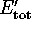and orbital angular momentum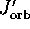are computed; if the new total energy is negative, then the new semimajor axis a' and eccentricity e' are calculated by using the newand; if the total energy is positive (that is, the binary is unbound) spatial velocities of each component are calculated.

During the CE stage, an effective spiral-inĀ of the binary components occurs. This complicated process (first introduced by Paczynsky, 1976) is not fully understood as yet, so we use the conventional energy consideration to find the binary system parameters after the CE by introducing a parameter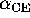measuring what fraction of the system's orbital energy (between the beginning and the end of the spiralling-in process) is transformed into the binding energy (gravitational minus thermal) of the ejected envelope. Thus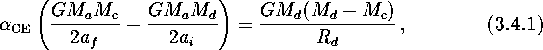where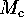is the mass of the core of the mass losing star of initial mass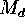and radius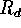(which is simply a function of the initial separationand the initial mass ratio), and no substantial mass growth is assumed for the accretor (see, however, Chevalier, 1993). The loweris, the closer the binary becomes after the CE stage. We will show below that the evolutionary scenario allows values ofover a wide range,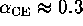-10.Next: ``Ecology'' of Magnetic Rotators Up: Evolutionary Scenarios of Binary Previous: Semi-major axis change

Mike E. Prokhorov
Sat Feb 22 18:38:13 MSK 1997